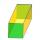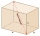# Diagonal + prism - math problems

#### Number of problems found: 34

• Prism diagonalThe body diagonal of a regular square prism has an angle of 60 degrees with the base, the edge length is 10 cm. What is the volume of the prism?
• Prism 4 sidesThe prism has a square base with a side length of 3 cm. The diagonal of the sidewall of the prism/BG/is 5 cm. Calculate the surface of this prism in cm square and the volume in liters
• PrismCalculate the volume of the rhombic prism. The prism base is a rhombus whose one diagonal is 47 cm, and the edge of the base is 28 cm. The edge length of the base of the prism and height is 3:5.The height of a regular quadrilateral prism is v = 10 cm, the deviation of the body diagonal from the base is 60°. Determine the length of the base edges, the surface, and the volume of the prism.Calculate the volume and surface area of a regular quadrangular prism 35 cm high and the base diagonal of 22 cm.
• Prism - boxThe base of prism is a rectangle with a side of 7.5 cm and 12.5 cm diagonal. The volume of the prism is V = 0.9 dm3. Calculate the surface of the prism.
• Four sided prismCalculate the volume and surface area of a regular quadrangular prism whose height is 28.6cm and the body diagonal forms a 50-degree angle with the base plane.
• PrismThe lenght, width and height of a right prism are 17, 11 and 11 respectively. What is the lenght of the longest segment whose endpoints are vertices of the prism?Calculate the volume (V) and the surface (S) of a regular quadrilateral prism whose height is 28.6 cm and the deviation of the body diagonal from the base plane is 50°.
• Trapezoidal prismCalculate the surface of the quadrilateral prism ABCDA'B'C'D 'with the trapezoidal base ABCD. The height of the prism is 12 cm; ABCD trapezoidal data: AB base length is 8 cm, CD base length is 3 cm, BC arm length is 4 cm, and AC diagonal length is 7 cm. L
• DiagonalDetermine the dimensions of the cuboid, if diagonal long 53 dm has an angle with one edge 42° and with another edge 64°.
• Angle of diagonalAngle between the body diagonal of a regular quadrilateral and its base is 60°. The edge of the base has a length of 10cm. Calculate the body volume.
• Total areaCalculate the total area (surface and bases) of a prism whose base is a rhombus which diagonals of 12cm and 18cm and prism height is 10 cm.
• Three faces of a cuboidThe diagonal of three faces of a cuboid are 13,√281 and 20 units. Then the total surface area of the cuboid is.
• LogsThe trunk diameter is 52 cm. Is it possible to inscribe a square prism with side 36 cm?
• Nice prismCalculate the cuboid's surface if the sum of its edges is a + b + c = 19 cm and the body diagonal size u = 13 cm.
• Regular hexagonal prismCalculate the volume of a regular hexagonal prism whose body diagonals are 24cm and 25cm long.
• Height of the roomGiven the floor area of a room as 24 feet by 48 feet and space diagonal of a room as 56 feet. Can you find the height of the room?
• Body diagonalCalculate the volume of a cuboid whose body diagonal u is equal to 6.1 cm. Rectangular base has dimensions of 3.2 cm and 2.4 cm
• A boxA box is 15 centimeters long, 4 centimeters wide, and 3 centimeters tall what is the diagonal S of the bottom side? What is the length of the body diagnol R?

Do you have an interesting mathematical word problem that you can't solve it? Submit a math problem, and we can try to solve it.

We will send a solution to your e-mail address. Solved examples are also published here. Please enter the e-mail correctly and check whether you don't have a full mailbox.

Please do not submit problems from current active competitions such as Mathematical Olympiad, correspondence seminars etc...